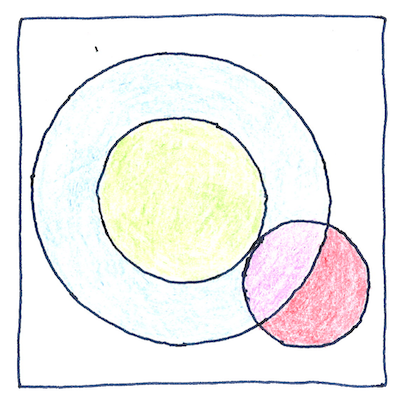# 1889

## The book of science

Tom Sharp

 Friedrich Paschen electromagnetism

## Paschen’s law

• Applying a voltage to electrodes
• at opposite ends of a gas-filled tube
• didn’t always make the tube glow.
• Scientists intending to generate
• an electric arc across a small gap sometimes got
• an arc following a long irregular path.
• Friedrich Paschen tested when the arc begins
• with different gas pressures
• and different distances between the electrodes
• showed that the breakthrough voltage
• is related to the pressure times the distance
• showed there’s an optimal distance and pressure
• for the minimum voltage for each kind of gas.

## Breakthrough voltage

• Townsend discharge doesn’t just happen.
• Each gas has its own ionization energy,
• the energy needed to dislodge one of its electrons.
• An electron must accelerate
• far enough without hitting anything
• to reach the ionization energy for a gas.
• If there isn’t enough space to accelerate
• or there are too many or two few atoms that it can hit
• then increasing the voltage might work.

## Optimal optimum, minimal minimum

• The lowest point of the Paschen curve
• shows the minimum voltage, which we find
• by setting the derivative of its equation to zero
• and solving for X. In real life, the trick
• is to determine the equation. Paschen
• discovered Paschen’s law empirically;
• that is, by experiment, which often requires
• that you simplify and eliminate noise. In Paschen’s
• case, we ignore the effect of outside light,
• and assume that one impact makes one ionization,
• that atoms recapturing electrons are not factors,
• and that electron production by the cathode
• is not affected by temperature or humidity.

One key difference between Egyptian geometry and Greek geometry is that the Greeks idealized the space that their geometry described. The Greek point has no dimension; the Greek line has no width; the Greek plane is perfectly flat and without thickness.

See also in The book of science:

Readings on wikipedia: